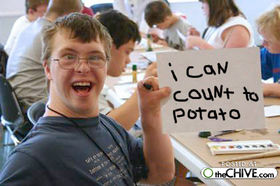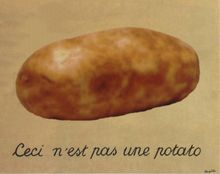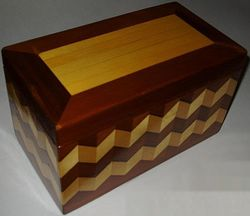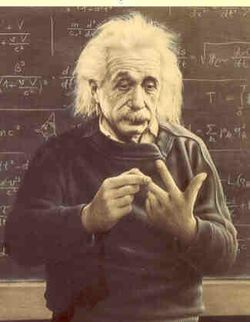# Counting to potato

Jump to navigation Jump to searchDespite being unable to perform simplistic algebraic calculations, this man can easily perform a mathematical operation that has puzzled the scientific community for generations. Nobody knows why.

Counting to potato is the act of arithmetically creating a sequence of numbers that ends with a potato, which is not a rational number but rather a tuber vegetable commonly eaten baked, boiled, or roasted. The problem has been a serious area of study among Western scientists for over a century, and is also referred to as the P equals NP problem, or $P=NP$.

Although no formal report on a successful count to potato has ever been deciphered, people afflicted with Down's Syndrome are often casually able to perform the feat, maddening mathematicians and physicists throughout human history.

## HistoryThe Magritte painting that referred to the difficulty of counting to a potato. When asked why he called it Ceci n'est pas une potato (Fr. "This is not a potato") he stated simply "Try boiling it. Actually, don't boil it really. It took me ages to get the shading right."

The problem first appears in print in Carl Friedrich Gauss's Demonstratio nova theorematis omnem functionem algebraicam rationalem integram unius variabilis in factores reales primi vel secundi gradus resolvi posse, which is Latin for something about Algebra. With the popularity of the potato spreading from King Louis XVI's court throughout Europe, the question of how to increase the production of potatos became a significant question for the mathematicians of the age.

Gauss, often referred to as Princeps mathematicorum posed the question Est slonum tuberosum a numerus? 

David Hilbert later included this as the 24th problem in his list of Hilbert's problems, but it was dropped prior to publishing, leaving the list at a total of 23.

## The Fibonacci Calculation

The problem was largely abandoned over the next several centuries, until famed mathematician Bernhard Riemann attempted to define potato using a recurrence relation. His theory was based upon Fibonacci's concepts in that that seeding the relationship F(n) = F(n-1)+F(n-2) with 0 and 1 and sending it to infinity would eventually transcend the mathematical plane and be incorporated into the material world, where it could be converted into potato using irrational roots and 3-dimensional geometric parameters. This attempt to count to potato was ultimately unsuccessful.

## Schrodinger's PotatoIf Schrodinger's theories had been correct, a simple wooden box like this could have made counting to potato a reality. Sadly, the search continues.

Erwin Schrodinger was one of the first physicists to tackle the concept of a count to potato, using a thought experiment based around perception. He wrote an infinite limit for the expression n+1, and placed it in a box sealed from the outside world, arguing that regardless of what expression was housed within the box, the inability to perceive it led to the creation of a limitless algorithm, including one terminating in potato. He failed to convince other leading physicists of the day, but a reworking of his theory became commonly accepted as an analogy for the Copenhagen interpretation of quantum dynamics.

## Albert EinsteinEinstein was a brilliant mathematician, but despite his best efforts, the formula needed to successfully count to potato eluded him

Einstein's attempt to count to potato was aided by Newton's invention of the derivative, the basis for calculus. Plotting the outlines of a potato onto a 2-dimensional vector graph, he attempted to derive the slope (m) of the potato, which he planned to incorporate into the formula lim(m-->p)[((2m^2)/m)-m], with p used as a variable for potato. While this was a unsuccessful thought experiment, his efforts to apply graphical analysis to the count to potato were seen as the most significant step forward so far.

Later in his life he returned to the problem in trying to find a link between the fields of mathematical knowledge and the physical world. Through numerous experiments he determined that the was a direct relationship between energy and mass. Understanding that energy is also directly related to power, and that power is knowledge, he determined that the spotaneous incorporalisation of a potato was possible with excessive knowledge. While he still was unable to create a potato using mathematical methodology only, the relationship between energy and mass, written algebraicly as $e=mc^{2}$was later published as his theory of relativity, and was responsible for the eventual creation of the Atomic bomb.

Einstein's actions remain the final large-scale attempt to count to potato, although small scale experiments have been performed as recently as 2009. Bill Gates has pledged \$1 000 000 to anyone successfully able to count to the famed vegetable, as has Richard Branson.

## The quater-tuberic number base

The quater-tuberic numeral system was first proposed by Donald Knuth in 1955, in a submission to Hilbert's problems. It is a non-standard positional numeral system which uses the tuberic number potato as its base. It was formulated to allow mathematicians to be able to count to the tuberic integer by using 0.5potatoi as a base, however due to the immutable nature of multiplication by potato, was limited to only being able to reach 7potato, the equivalent of 1310, and anything beyond that being referred to as simply more than 7potato. This also had the difficulty of having potato as having multiple values, and therefore being impractical as a counting method. The numerals available were counted as such:

• 1, potato, 2, potato, 3, potato, 4, 5, potato, 6, potato, 7, potato, more

Due to its obvious limitations, this was dismissed by the majority of the mathematical community as pseudo-mathematics.

## Current Implications

The problem of counting to potato has been included in the "Millennium Prize Problems" and is often referred to in shorthand as NP = P (the numerical value potato is equal to a potato). It is considered that the solution to this would be one of the most fundamental leaps forward in mathematical knowledge.

|If P = NP, then the world would be a profoundly different place than we usually assume it to be. There would be no special value in "creative leaps," no fundamental gap between solving a problem and recognizing the solution once it's found. Everyone who could appreciate a symphony would be Mozart; everyone who could follow a step-by-step argument would be Gauss...
~ Scott Aaronson, MIT